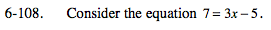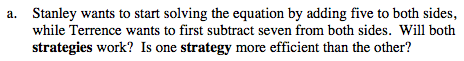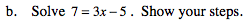### Home > MC2 > Chapter 6 > Lesson 6.2.4 > Problem6-108

6-108.Solving the equation ultimately involves isolating x to determine its value.
If both strategies are working towards isolating x through different methods, will both strategies work?

Does one strategy require less work to isolate x?
Is the strategy of adding 5 to both sides rather than subtracting 7 a more efficient and direct method?7 = 3x − 5
+5 +5

$\frac{12}{3}=\frac{3x}{3}$

x = 4